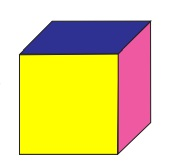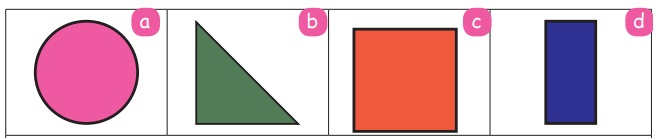Home | | Maths 3rd Std | Diagonal

# Diagonal

Diagonal is the line joining opposite corners of a geometrical shape.

Diagonal

Diagonal is the line joining opposite corners of a geometrical shape.

Observe the corners of the square.The line joining opposite corners of a square is called the diagonal of the square.

A square has two diagonals.

Diagonals of a cube:

A Cube has six square faces. Each square has two diagonals.

Diagonals in the faces = 6 × 2 = 12.

Diagonals in the inner sides of 4 corners = 4.

Total number of diagonals of a cube = 12 + 4 = 16.Draw the diagonals for the given rectangle.How many diagonals will be there in a cuboid? 16 .

Match the properties of 2D shapes by observing their sides and corners (vertices).1. Opposite sides are equal. (d)

2. There are no sides and corners. (a)

3. Sides may or may not be equal. (b)

4. All the four sides are equal. (c)

Tags : Geometry | Term 3 Chapter 1 | 3rd Maths , 3rd Maths : Term 3 Unit 1 : Geometry
Study Material, Lecturing Notes, Assignment, Reference, Wiki description explanation, brief detail
3rd Maths : Term 3 Unit 1 : Geometry : Diagonal | Geometry | Term 3 Chapter 1 | 3rd Maths